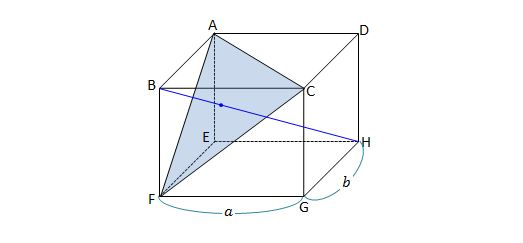Geometry

# 3D Section Formula

What are the coordinates of $$P$$ which divide the line segment joining the points $$A=(-4,4,1)$$ and $$B=(4,-4,2)$$ in the ratio $$3:2 ?$$

Let $$A ( 4, 3, 5 )$$ and $$B ( 2, 6 , 0)$$ be two fixed points. Find the position vector of the point which lies on the line segment $$\overline{AB}$$ and internally divides it in the ratio $$7 : 8.$$The above rectangular cuboid has base dimensions $$a=12$$ and $$b=8.$$ If the centroid $$I$$ of triangle $$ACF$$ internally divides the diagonal $$\overline{BH}$$ of the rectangular cuboid in the ratio $$BI:IH=1:m,$$ what is the value of $$m?$$

Suppose three vertices of a parallelogram are $O=(0,0,0), A=(1,5,6), B=(-1,-5,5),$ where $$\overline{OA}$$ and $$\overline{OB}$$ are adjacent sides. If $$C$$ is the final vertex of the parallelogram, then what are the coordinates of $$C ?$$

Consider a triangle $$ABC$$ such that the centroid of of the triangle is $$G=(5,-2,3)$$ and the midpoint of $$\overline{AB}$$ is $$P=\left(1,\frac{1}{2},\frac{1}{2}\right).$$ What are the coordinates of the point $$C ?$$

×